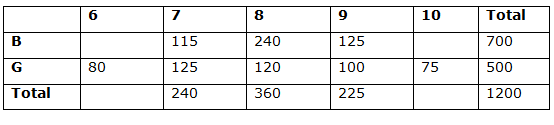# “The Knowledge Library”

Knowledge for All, without Barriers……….

# Data Interpretation-11

Directions (1 – 5): Study the following information carefully and answer the questions given below.

Pie graphs show the % of applications received from various states in 2017 and 2018

1) What is the difference between the no. of applications received from A and C in 2017 and B, C and F in 2018?

a) 1568

b) 1398

c) 2076

d) 1386

e) None of these

2) If 36% of total no. of applications are rejected in 2017 and no. of applications rejected from D is 194, what is the approximate% of valid applications from D to total no. of applications?

a) 84%

b) 65.44%

c) 52.02%

d) 79.54%

e) None of these

3) What is the ratio of the no. of applications from A and E in 2017 to A, B, D in 2018 if 420 applicants apply from state A instead of E both the years?

a) 84:123

b) 64:161

c) 75:81

d) 72:121

e) None of these

4) If 20% of applicants are male in state D in 2017 and 40% of applicants are female in state C in 2017. The no. of female applicants from D is how much more/less than the no. of male applicants from C?

a) 3278

b) 3192

c) 2059

d) 2166

e) None of these

5) In 2018, 25% of applicants from state D and 35% of applicants from state E apply from state A, the no. of applicants from state A?

a) 7684

b) 6554

c) 6678

d) 7379

e) None of these

Caselet

Directions (6 – 10): Study the following information carefully and answer the questions given below?

A total of 1200 students are studying in a school. There are 5 classes in the school from 6 to 10. The ratio of the number of boys to girls is 7: 5. In class 8, 2/3rd students are boys. 20% of students belong to class 7. 80 is the number of girls students in class 6. A total of 360 students are in class 8. Number of boys in class 9 is 125% of girls in that class where the number of girls in class 9 is 20% of total number of girls. Class 10 has 75 girls. The ratio of the number of boys to girls in class 7 is 23:25

6) What is the ratio between the no. of boys in 8th and the no. of girls in 7th?

a) 48:25

b) 45: 28

c) 24: 35

d) 28: 37

e) None of these

7) If the total no. of students in class 6 is 200, the no. of boys from 6, 7, 8 together form what % of total no. of boys?

a) 48.55%

b) 45.78%

c) 67.85%

d) 57.76%

e) None of these

8) What is the difference between the average of boys from 7, 8, 9 together and the average of girls from 6, 7, 8 together?

a) 48.25

b) 51.66

c) 24.35

d) 58.97

e) None of these

9) If the no. of boys in class 10 is 100, the difference between class 10 boys and class 9 boys are how much less than the difference between class 8 girls and class 6 girls?

a) 15

b) 40

c) 25

d) 45

e) None of these

10) What is the total number of students in classes 7, 8, 9?

a) 925

b) 540

c) 746

d) 825

e) None of these

Directions (1-5) :

A&C in 2017 = 35% 11400 = 3990

B, C, F in 2018 = 32% 16800 = 5376

Diff = 1386

36% of 11400 = 4104

No. of valid app = 11400 – 4104 = 7296

Total no. of app from D = 35% of 11400 = 3990

Total no. of valid app from D = 3990 – 194 = 3796

3796 / 7296 * 100 = 52.02%

30% of 11400 = 3420

3420 + 420 = 3840 – – – 1

55% of 16800 = 9240

9240 + 420 = 9660 – – – 2

Now, 3840: 9660 = 64: 161

80% of 35% of 11400 – 60% of 15% of 11400

3192 – 1026 = 2166

25% of 16% of 16800 + 35% of 25% of 16800 + 27% of 16800

672 + 1470 + 4536 = 6678

Directions  (6 – 10):Required ratio

= > 240 : 125 = 48:25

6th boys = 200 – 80 = 120

6, 7, 8 boys = 475

Required percentage = 475 / 700 * 100 = 67.85%

Required difference

= > 480 / 3 – 325 / 3 = 155 / 3 = 51.66

Required difference

= > (125 – 100) – (120 – 80) = 15

The total number of students in classes 7, 8, 9

= > 240 + 360 + 225 = 825